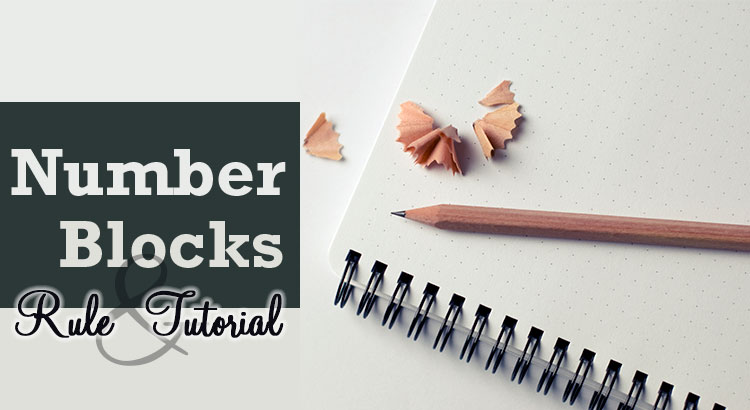# Rule and Tutorial of NumberBlocks

## Introduction

NumberBlocks is a puzzle game which we have to determine the place of numbers by using given hints under some rules. Here we explain the rule of NumberBlocks with size 4×4 in detail for those who plays it for the first time. Then we demonstrate the solving process of an example puzzle.

In explanations, we call a horizontal sequence of boxes row and a vertical sequence of boxes column. We describe a position of a box as (1,1) using a row and a column number. For example, we call the colored box in the following image box–(2,3).## Rule

There are three rules for NumberBlocks. Please remember them all.

1. Each row contains each digit of 1–4 exactly once.
2. Each column contains each digit of 1–4 exactly once.
3. Each region surrounded by thick lines contains each digit of 1–4 exactly once.

Now we explain each rule in detail by using the example puzzle following.### Rule 1

Each row contains each digit of 1–4 exactly once. This means that we cannot place same numbers in a row. In the example, a number 2 is placed in box–(2,4). So we cannot place another number 2 in box–(2,1), box–(2,2) and box–(2,3).### Rule 2

Each column contains each digit of 1–4 exactly once. This means that we cannot place same numbers in a column. In the example, a number 2 is placed in box–(2,4). So we cannot place another number 2 in box–(1,4), box–(3,4) and box–(4,4).### Rule 3

Each region surrounded by thick lines contains each digit of 1–4 exactly once. This means that we cannot place same numbers in a region. In the example, we can see four regions. Box–(1,1), box–(1,2), box–(2,1) and box–(3,1) forms one regions. A number 3 is placed in box–(3,1). Then we cannot place another number 1 in box–(1,1), box–(1,2) and box–(2,1).## Tutorial

Here, we demonstrate a solving process of the example puzzle. This is not the only process to solve. We hope you will find other processes.

### Process 1

A basic technique to solve NumberBlocks is to find boxes in which a specific number should be placed. For that, we focus on given hints. First, we focus on a number 1 of box–(3,1). Then we fill boxes in which we cannot place a number 1 with a color by following rules.Then, in each region, we have several boxes in which we can place a number 1. So we cannot decide which is the right box to place a number 1.

Next, we focus on a number 2 of box–(2,4). Then we fill boxes in which we cannot place a number 2 with a color by following rules.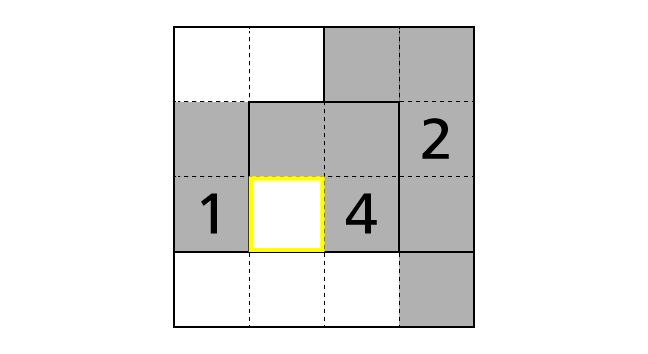This time, we notice that there is a box (surrounded by yellow lines) that number 2 is the only choice to be placed in. Because, by rule 3, we have to place a number 2 exactly once in the middle region. But, by rule 1, we cannot place a number 2 in box–(2,2) and box–(2,3). So box–(3,2) is the only box that we can place a number 2 in.

Now we decided the place of a number.### Process 2

Next, let’s focus on row 3.By rule 1, we know that each row contains each digit of 1–4 exactly once. But number 1, 2 and 4 are already placed in row 3. So box–(3,4) is the only box that we can place a number 3 in.### Process 3

Please recall that we placed a number 2 in box–(3,2) in process 1. Then we can guess that this decrease the count of boxes in which we can place a number 2.
So let’s fill boxes in which we cannot place a number 2 with a color by following rules again.By rule 3, we have to place a number 2 exactly once in the top left region. Now we notice that box–(1,1) is the only box that we can place a number 2 in. Let’s place a number.### Process 4

Next, let’s fill boxes in which we cannot place a number 2 with a color again.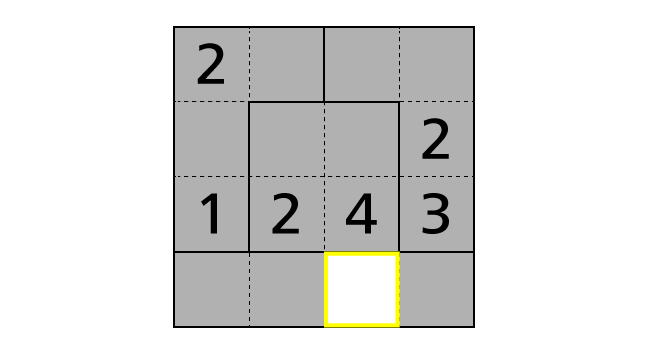By rule 3, we have to place a number 2 exactly once in the bottom region. Now we notice that box–(4,3) is the only box that we can place a number 2 in. Let’s place a number.### Process 5

Next, we focus on number 4. Then we fill boxes in which we cannot place a number 4 with a color by following rules.Then, by rule 3, we notice that box–(1,4) is the only box that we can place a number 4 in. Let’s place a number.### Process 6

Next, we focus on the top right region and column 4. Then, by rule 3, we notice that box–(1,3) is the only box that we can place a number 1 in. Moreover, by rule 2, we notice that box–(4,4) is the only box that we can place a number 1 in.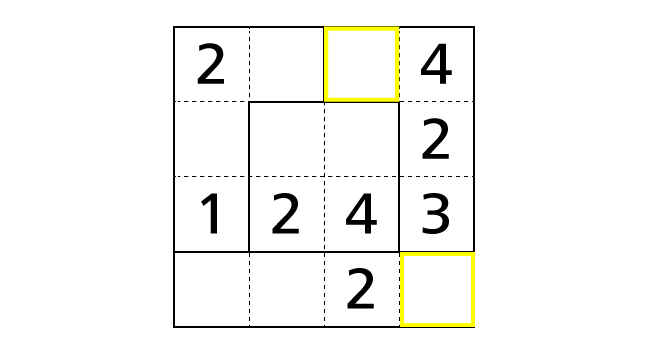Now let’s place numbers.### Process 7

Next, we focus on row 1 and column 3. Then, by rule 1, we notice that box–(1,2) is the only box that we can place a number 3 in. Moreover, by rule 2, we notice that box–(2,3) is the only box that we can place a number 3 in.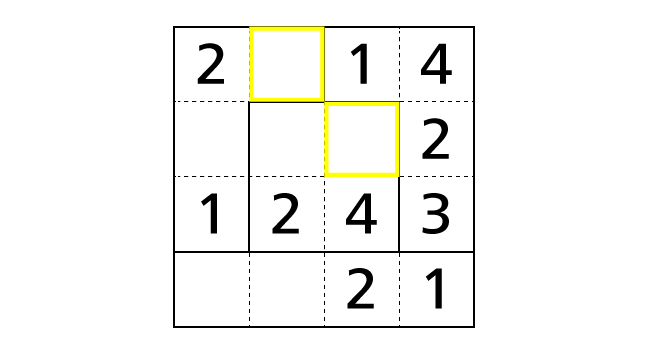Now let’s place numbers.### Process 8

Next, we focus on the top left region. Then, by rule 3, we notice that box–(2,1) is the only box that we can place a number 4 in.Now let’s place a number.### Process 9

Next, we focus on row 2 and column 1. Then, by rule 1, we notice that box–(2,2) is the only box that we can place a number 1 in. Moreover, by rule 2, we notice that box–(4,1) is the only box that we can place a number 3 in.Now let’s place numbers.### Process 10

Next, we focus on row 4. Then, by rule 1 or by rule 3, we notice that box–(4,2) is the only box that we can place a number 4 in. Finally, we reached the answer.## Get Started

Most recently uploaded NumberBlocks puzzles are available on NumberBlocks.
Previous NumberBlocks puzzles are available on NumberBlocks Archives.